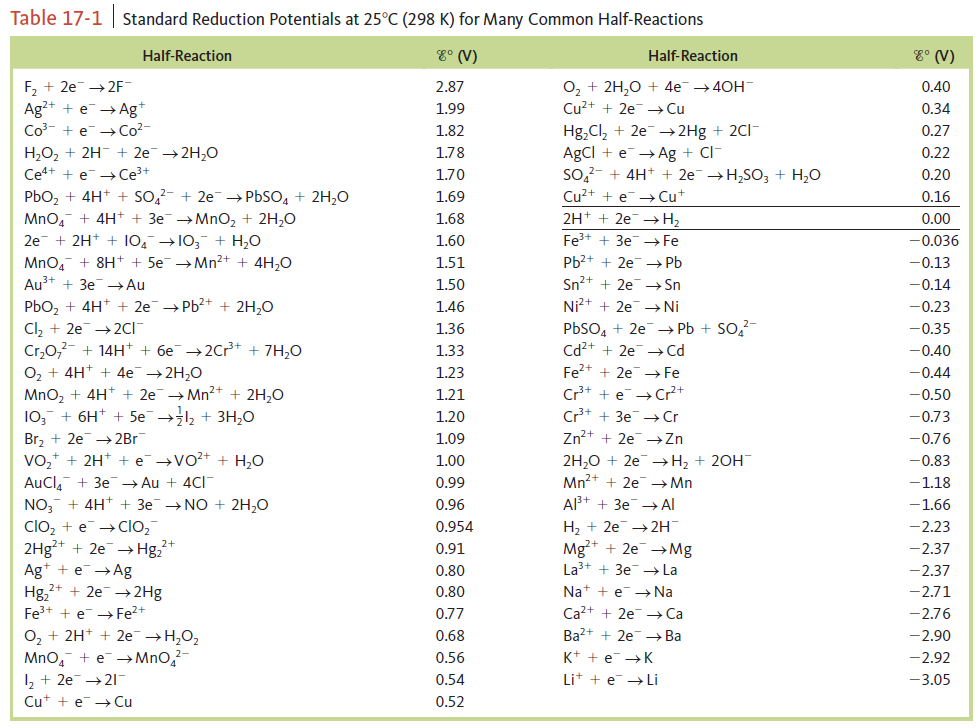# Problem: Calculate E° values for the following cells. Which reactions are spontaneous as written (under standard conditions)? Balance the equations that are not already balanced. Standard reduction potentials are found in Table 17‑1.b. Au3+ (aq) + Ag (s) → Ag + (aq) + Au (s)

⚠️Our tutors found the solution shown to be helpful for the problem you're searching for. We don't have the exact solution yet.

###### Problem Details

Calculate E° values for the following cells. Which reactions are spontaneous as written (under standard conditions)? Balance the equations that are not already balanced. Standard reduction potentials are found in Table 17‑1.

b. Au3+ (aq) + Ag (s) → Ag + (aq) + Au (s)